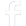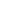#### WhatsApp Quick Chat#### Get Instant Call Back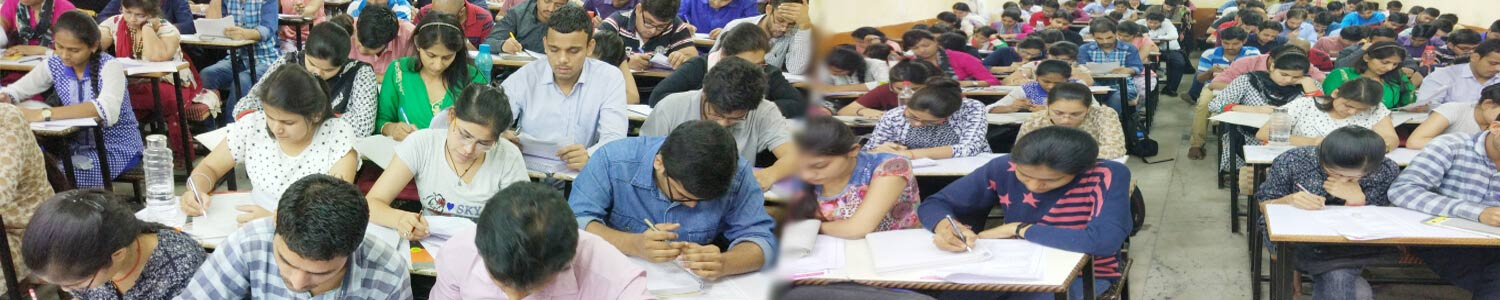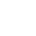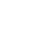## Offers## News & Events# IIT JAM Mathematics Syllabus And Reference Books

##### JAM: MATHEMATICS

Sequences and Series of Real Numbers: Sequences and series of real numbers, Convergent and divergent sequences, bounded and monotone sequences, Convergence criteria for sequences of real numbers, Cauchy sequences, absolute and conditional convergence; Tests of convergence for series of positive terms – comparison test, ratio test, root test; Leibnitz test for convergence of alternating series.

##### Functions of one Variable:

Functions of One Variable: limit, continuity, differentiation, Rolle's Theorem, Mean value theorem. Taylor's theorem, Maxima and minima.

Functions of Two Real Variables: limit, continuity, partial derivatives, differentiability, maxima and minima. Method of Lagrange multipliers, Homogeneous functions including Euler's theorem.

Integral Calculus: Integration as the inverse process of differentiation, definite integrals and their properties, Fundamental theorem of integral calculus. Double and triple integrals, change of order of integration. Calculating surface areas and volumes using double integrals and applications. Calculating volumes using triple integrals and applications.

Differential Equations: Ordinary differential equations of the first order of the form y'=f(x,y). Bernoulli's equation, exact differential equations, integrating factor, Orthogonal trajectories, Homogeneous differential equations-separable solutions, Linear differential equations of second and higher order with constant coefficients, method of variation of parameters. Cauchy-Euler equation.

Vector Calculus: Scalar and vector fields, gradient, divergence, curl and Laplacian. Scalar line integrals and vector line integrals, scalar surface integrals and vector surface integrals, Green's, Stokes and Gauss theorems and their applications.

Group Theory: Groups, subgroups, Abelian groups, non-abelian groups, cyclic groups, permutation groups; Normal subgroups, Lagrange's Theorem for finite groups, group homomorphisms and basic concepts of quotient groups (only group theory).

Linear Algebra: Vector spaces, Linear dependence of vectors, basis, dimension, linear transformations, matrix representation with respect to an ordered basis, Range space and null space, rank-nullity theorem; Rank and inverse of a matrix, determinant, solutions of systems of linear equations, consistency conditions. Eigenvalues and eigenvectors. Cayley-Hamilton theorem. Symmetric, skew-symmetric, hermitian, skew-hermitian, orthogonal and unitary matrices.

Real Analysis: Interior points, limit points, open sets, closed sets, bounded sets, connected sets, compact sets; completeness of R, Power series (of real variable) including Taylor's and Maclaurin's, domain of convergence, term-wise differentiation and integration of power series.

##### JAM: MATHEMATICAL STATISTICS (MS)

The Mathematical Statistics (MS) test paper comprises of Mathematics (40% weightage) and Statistics (60% weightage).

MATHEMATICS Sequences and Series: Convergence of sequences of real numbers, Comparison, root and ratio tests for convergence of series of real numbers.

Differential Calculus: Limits, continuity and differentiability of functions of one and two variables. Rolle's theorem, mean value theorems, Taylor's theorem, indeterminate forms, maxima and minima of functions of one and two variables.

Integral Calculus: Fundamental theorems of integral calculus. Double and triple integrals, applications of definite integrals, arc lengths, areas and volumes.

Matrices: Rank, inverse of a matrix. Systems of linear equations. Linear transformations, eigenvalues and eigenvectors. Cayley-Hamilton theorem, symmetric, skew-symmetric and orthogonal matrices.

Differential Equations: Ordinary differential equations of the first order of the form y' = f(x,y). Linear differential equations of the second order with constant coefficients.

##### STATISTICS

Probability: Axiomatic definition of probability and properties, conditional probability, multiplication rule. Theorem of total probability. Bayes' theorem and independence of events.

Random Variables: Probability mass function, probability density function and cumulative distribution functions, distribution of a function of a random variable. Mathematical expectation, moments and moment generating function. Chebyshev's inequality.

Standard Distributions: Binomial, negative binomial, geometric, Poisson, hypergeometric, uniform, exponential, gamma, beta and normal distributions. Poisson and normal approximations of a binomial distribution.

Joint Distributions:Joint, marginal and conditional distributions. Distribution of functions of random variables. Product moments, correlation, simple linear regression. Independence of random variables.

Sampling distributions: Chi-square, t and F distributions, and their properties.

Limit Theorems: Weak law of large numbers. Central limit theorem (i.i.d. with finite variance case only).

Estimation: Unbiasedness, consistency and efficiency of estimators, method of moments and method of maximum likelihood. Sufficiency, factorization theorem. Completeness, Rao-Blackwell and Lehmann- Scheffe theorems, uniformly minimum variance unbiased estimators. Rao-Cramer inequality. Confidence intervals for the parameters of univariate normal, two independent normal, and one parameter exponential distributions.

Testing of Hypotheses: Basic concepts, applications of Neyman-Pearson Lemma for testing simple and composite hypotheses. Likelihood ratio tests for parameters of univariate normal distribution.

## Offers